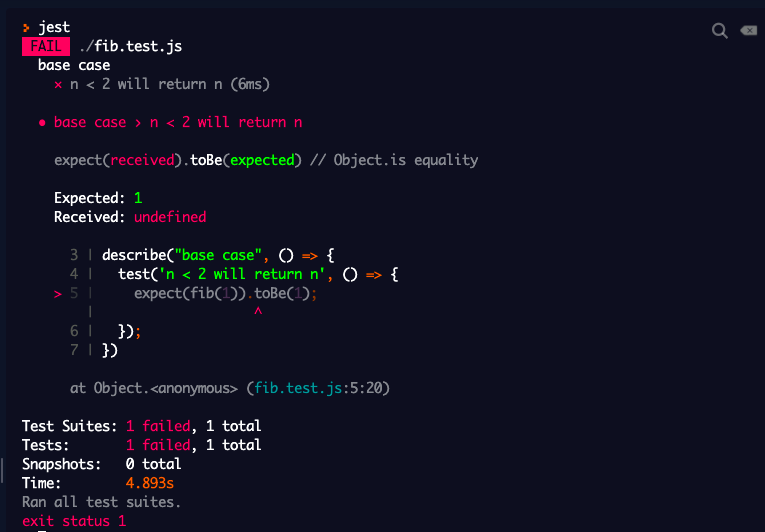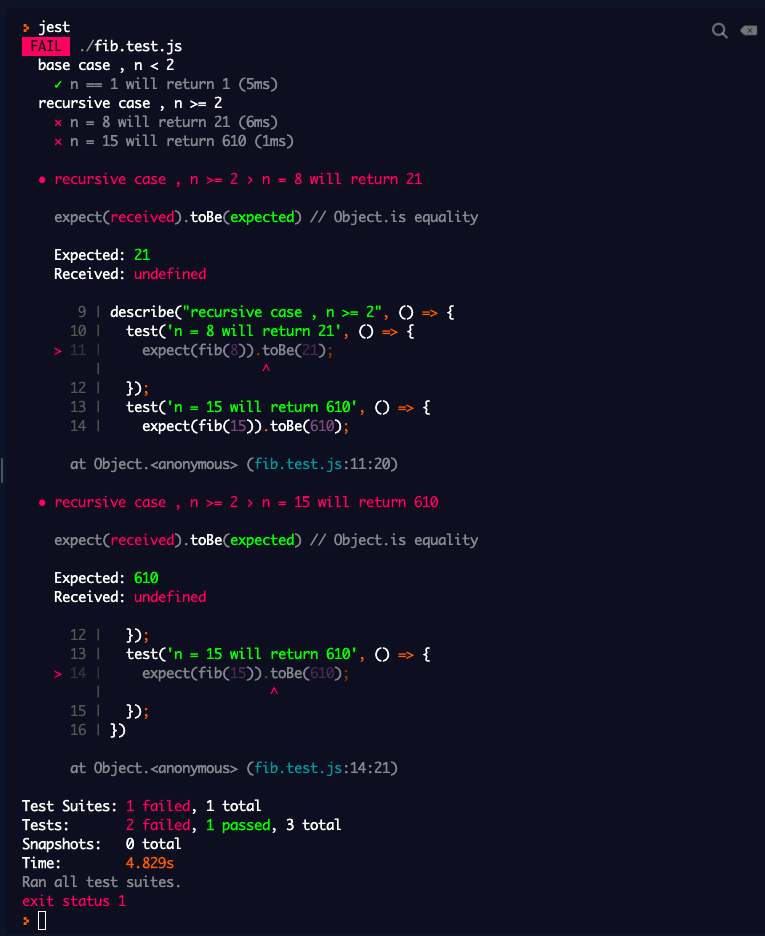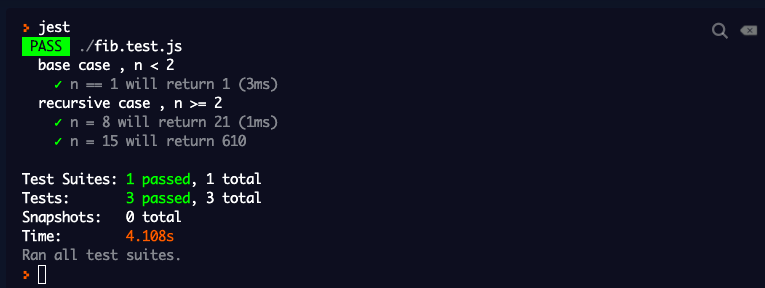← All Articles

# Red, Green, Refactor

— Written byTDD is tricky and we need to be smart to get the maximum throughput of what TDD offers. TDD, done wrong could waste hours and will not look as effective as we would have thought. This is where the Red, Green, Refactor approach comes to save us from the pain.

Red, Green, Refactor is a TDD approach or framework that the developers use to build the test suite first, write the implementation code, and refactor the code once the test suite passes.

According to Computer Scientist Robert C Martin who advocates TDD, we can write effective code only when there is a test case to pass and the only time we have the time to write tests is before writing the implementation code. The feasible way to do so is to use the Red, Green, Refactor approach.

## What are they?

We can compartmentalize writing code into 3 segments as the title suggests.

Red - Write a test suite without the implementation code, making it fail.

Green - Now, we can write the implementation code, just so the test suite passes. Nothing more, nothing less.

Refactor - After the test suite passes, we can look for ways to optimize.

…rinse & repeat. This happens until we have a fully functional implementation code.

Robert C. Martin (“Uncle Bob”) provides a concise set of rules for practicing TDD.

1. Write production code only to pass a failing unit test.
2. Write no more of a unit test than sufficient to fail (compilation failures are failures).
3. Write no more production code than necessary to pass the one failing unit test.

## How is it done?

We can see how it’s done by looking at an implementation of the Fibonacci problem with recursion. We’ll use the Jest playground.

### Iteration 1

We have two JavaScript files, one is `fib.js` to contain the implementation code, `fib.test.js` to contain the test suites. As an overkill, you can start without the function defined in `fib.js`. Let me keep the post as short as possible.

#### Red

Since we are solving the problem with recursion, we need to first define our base case first. Which is, if n is lesser than 2, we need to return n.

Let’s first write the test suite for the base case, which will be the following,

``````const fib = require("./fib");

describe("base case , n < 2", () => {
test("n = 1 will return 1", () => {
expect(fib(1)).toBe(1);
});
});
``````

We expect this to fail since we don’t have any implementation code.#### Green

We now need to write the implementation code for the base case. Remember, only the code that’s needed to make our test suite pass.

``````function fib(n) {
if (n < 2) {
return n;
}
}
module.exports = fib;
``````

Now, this code satisfies our test suite. What’s next? Let’s look at refactoring the above code.

#### Refactor

From the above implementation code, we don’t have anything much to refactor so let’s move to Iteration 2.

### Iteration 2

#### Red

Now we have our base case, let’s look at the next step which is to write the recursive code. Let’s extend our test suite to test the recursive case.

``````describe("recursive case , n >= 2", () => {
test("n = 8 will return 21", () => {
expect(fib(8)).toBe(21);
});
test("n = 15 will return 610", () => {
expect(fib(15)).toBe(610);
});
});
``````

Now, that we extended our test suite, let’s see the failing test case result which is as follows,As you have guessed, this is because, in our implementation code, we check if n is lesser than 2 and return n. It doesn’t currently handle the case where n is greater than or equal to 2.

#### Green

We’ll now write the implementation code so that our test suite passes.

``````function fib(n) {
if (n < 2) {
return n;
} else {
return fib(n - 1) + fib(n - 2);
}
}
module.exports = fib;
``````

With recursion, we have written the implementation code to handle the case where n >= 2. We’ll now have Green, where our test suite passes.#### Refactor

What could we possibly do here to refactor the above code? Not much, but as a cosmetic update, we can remove the unwanted braces and the else part since we are returning in the if part. After refactoring, our code will look like the following,

``````function fib(n) {
if (n < 2) return n;

return fib(n - 1) + fib(n - 2);
}
module.exports = fib;
``````

### Iteration 3

Well, there’s no Iteration 3 since we have a fully functional module. So, it ends here.

## Conclusion

This approach might look time-consuming at first, but once we get a hold of it, it can be employed to write effective testable code that will make everyone’s life easier, while building a more robust solution than you otherwise would have.

Ok, bye!Up next

SwiftUI + WidgetKitSkcript /svr/red-green-refactor/ /svrmedia/heroes/red-green-refactor-2x.jpg

We collaborate with ambitious brands and people.

Talk to a consultant →